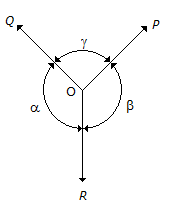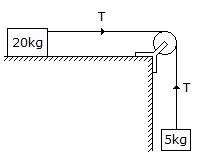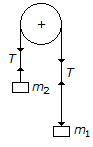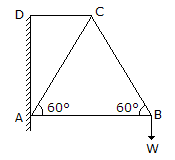Courses

# Test: Engineering Mechanics - 1

## 25 Questions MCQ Test Engineering Mechanics | Test: Engineering Mechanics - 1

Description
This mock test of Test: Engineering Mechanics - 1 for Mechanical Engineering helps you for every Mechanical Engineering entrance exam. This contains 25 Multiple Choice Questions for Mechanical Engineering Test: Engineering Mechanics - 1 (mcq) to study with solutions a complete question bank. The solved questions answers in this Test: Engineering Mechanics - 1 quiz give you a good mix of easy questions and tough questions. Mechanical Engineering students definitely take this Test: Engineering Mechanics - 1 exercise for a better result in the exam. You can find other Test: Engineering Mechanics - 1 extra questions, long questions & short questions for Mechanical Engineering on EduRev as well by searching above.
QUESTION: 1

Solution:
QUESTION: 2

Solution:
QUESTION: 3

### The centre of gravity of a hemisphere lies at a distance of 3r / 8 from its base measured along the vertical radius.

Solution:
QUESTION: 4The above figure shows the three coplaner forces P, Q and R acting at a point O. If these forces are in equilibrium, then

Solution:
QUESTION: 5

Moment of inertia of a rectangular section having width (b) and depth (d) about an axis passing through its C.G. and parallel to the depth (d), is

Solution:
QUESTION: 6

A smooth cylinder lying on a __________ is in neutral equilibrium.

Solution:
QUESTION: 7

The polygon law of forces states that if a number of forces, acting simultaneously on a particle, be represented in magnitude and direction by the sides a polygon taken in order, then their resultant is represented in magnitude and direction by the closing side of the polygon, taken in opposite direction.

Solution:
QUESTION: 8

Varingon's theorem of moments states that if a number of coplaner forces acting on a particle are in equilibrium, then

Solution:
QUESTION: 9

The velocity of a particle (v) moving with simple harmonic motion, at any instant is given by(where r = Amplitude of motion, and y = Displacement of the particle from mean position.)

Solution:
QUESTION: 10

A block ot mass 20 kg lying on a rough horizontal plane is connected by a light string passing over a smooth pulley to another mass 5 kg, which can move freely in the Vertical direction, as shown in the below figure. The tension in the string will __________ with the increase in coefficient of friction.Solution:

Coefficient of friction = m1g/(m+ m2).
= (5/25)g.
Tension = m1m2g/(m1+m2).
= 15g/4.
So both +ve so increase.

QUESTION: 11

One end of a helical spring is fixed while the other end carries the load W which moves with simple harmonic motion. The frequency of motion is given by(where δ = Deflection of the spring.)

Solution:

Time = 2π(√δ/g).
frequency = 1/time.
= 1/2π(√g/δ)

QUESTION: 12

The angle of inclination with the vertical is __________ if the cyclist is running at a faster speed than that when he is running at a slower speed.

Solution:
QUESTION: 13

The range of projectile(R) on an upward inclined plane is

Solution:
QUESTION: 14

The centre of gravity of a right circular solid cone is at a distance of __________ from its base, measured along the vertical axis.(where h = Height of a right circular solid cone.)

Solution:
QUESTION: 15

In actual machines, mechanical advantage is __________ velocity ratio.

Solution:
QUESTION: 16

The radius of gyration is the distance where the whole mass (or area) of a body is assumed to be concentrated.

Solution:
QUESTION: 17

If three coplaner forces acting on a point are in equilibrium, then each force is proportional to the sine, of the angle between the other two.

Solution:
QUESTION: 18

A rubber ball is dropped from a height of 2 m. If there is no loss of velocity after rebounding, the ball will rise to a height of

Solution:
QUESTION: 19

In the shown figure, the tension (T) in the string will beSolution:
QUESTION: 20

When the spring of a watch is wound, it will possess

Solution:
QUESTION: 21

In a framed structure, as shown in the below figure, the force in the member CD is tensile in nature.Solution:
QUESTION: 22

An irregular body may have more than one centre of gravity.

Solution:
QUESTION: 23

The rate of doing work is known as

Solution:
QUESTION: 24

In a wormed geared pulley block, if the number of teeth on the worm wheel is doubled, then its velocity ratio is also doubled.

Solution:
QUESTION: 25

Moment of inertia of a circular section about its diameter (d) is

Solution: